## Solve division problems without remainders using the area model

Enter the password to open this PDF file:## Division using the Area Model

These lessons, with videos, examples, and solutions help Grade 4 students learn how to solve division problems without remainders using the area model.

Related Pages Lesson Plans and Worksheets for Grade 4 Lesson Plans and Worksheets for all Grades More Lessons for Grade 4 Common Core For Grade 4

Common Core Standards: 4.NBT.6, 4.OA.3

Topic E: Division of Tens and Ones with Successive Remainders

New York State Common Core Math Grade 4, Module 3, Lesson 20 Grade 4, Module 3, Lesson 20 Worksheets

The following figure shows an example of division using the area model. Scroll down the page for more examples and solutions.NYS Math Grade 4, Module 3, Lesson 20 Concept Development Problem 1: Decompose 48 ÷ 4 from whole to part. Problem 2: Decompose 96 ÷ 4 from whole to part.

NYS Math Grade 4, Module 3, Lesson 20 Problem Set

• Alfonso solved a division problem by drawing an area model. a. Look at the area model. What division problem did Alfonso solve? b. Show a number bond to represent Alfonso’s area model. Start with the total and then show how the total is split into two parts. Below the two parts, represent the total length using the distributive property and then solve.
• Solve 45 ÷ 3 using an area model. Draw a number bond and use the distributive property to solve for the unknown length.
• Solve 60 ÷ 4 using an area model. Draw a number bond to show how you partitioned the area, and represent the division with a written method.
• Solve 72 ÷ 4 using an area model. Explain, using words, pictures, or numbers, the connection of the distributive property to the area model.
• Solve 72 ÷ 6 using an area model and the standard algorithm.

NYS Math Module 3 Grade 4 Lesson 20 Homework

• Maria solved the following division problem by drawing an area model. a. Look at the area model. What division problem did Maria solve? b. Show a number bond to represent Maria’s area model. Start with the total and then show how the total is split into two parts. Below the two parts, represent the total length using the distributive property and then solve.
• Solve 42 ÷ 3 using an area model. Draw a number bond and use the distributive property to solve for the unknown length.
• Solve 96 ÷ 6 using an area model and the standard algorithm.## Area Model Division

What is the area model division, introducing area model division, benefits of using area model division, solved examples, practice problems, frequently asked questions.

When they first learn how to divide numbers in school, most people are taught the traditional method of finding a common denominator and then doing long division.

However, there’s an alternative that can make the problem of dividing fractions or other numbers much easier. It’s called the area model method. Let’s learn more about it!

The area of a shape is the space occupied by the shape .

If a rectangle has a length equal to 32 units and a width equal to 23 units, then we can find its area by calculating the product $32 \times 23$.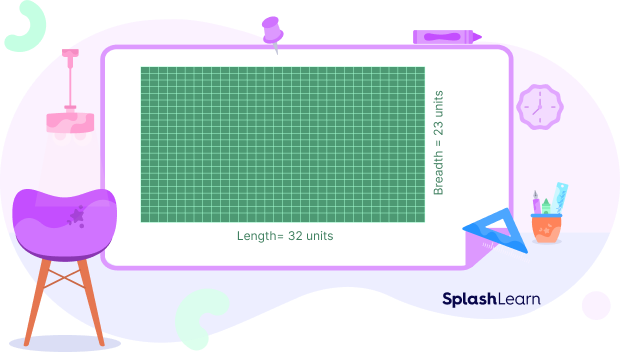In other words, when we consider the product $32 \times 23$ geometrically, it can be interpreted as the area of a rectangle of length 32 units and width 23 units.

Similarly, we can geometrically interpret a division problem, say $555 \div 15$, as the missing dimension of a rectangle of area 555 square units and one side length 15 units.• We can divide this rectangle into several small rectangles. Then, we can calculate the length of each small rectangle and add them together to find the length of the large rectangle.
• First, consider a small rectangle of width 15 units and length 20 units. The area of this rectangle is 300 square units. So the area of the rest of the rectangle is $555$ $–$ $300 = 255$ square units.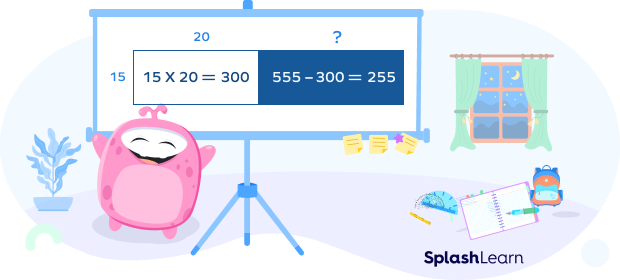Now, we have an area of 255 square units left. Since $15 \times 10 = 150$, another rectangle of width 15 units and length 10 units can be created.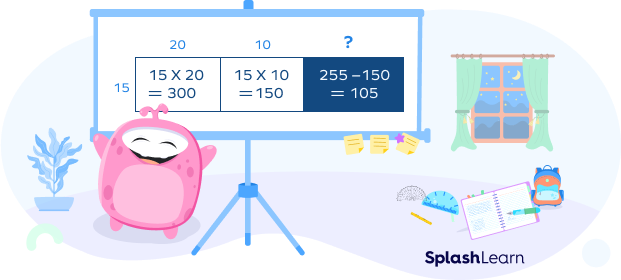We are now left with an area of $255$ $–$ $150 = 105$ square units. As $15 \times 7 = 105$, the shaded rectangle has a width of 15 units and a length of 7 units.

Thus, the length of the large rectangle is $20 + 10 + 7 = 37$ units. Therefore, $555 \div 15 = 37$.

## Related Games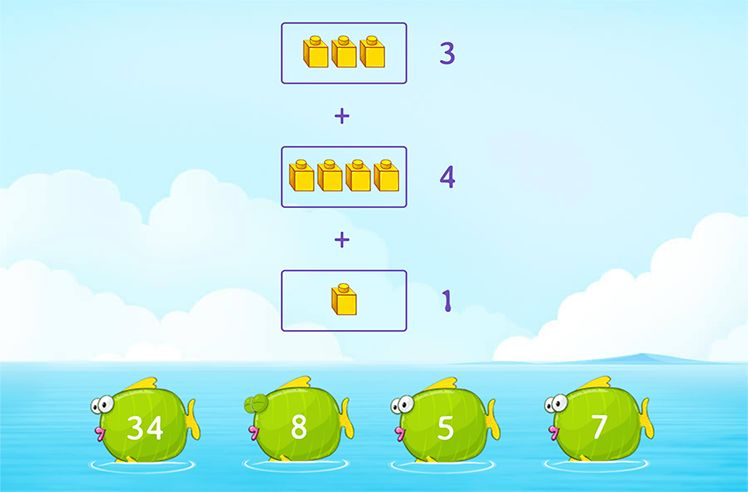Let’s learn how to use area model division to divide 825 by 5.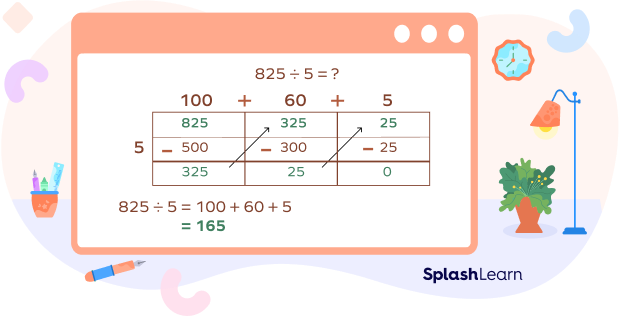Step 1: Let’s start by breaking 825 into 500, 300, and 25, which are fairly easy to divide by 5.

Step 2: Now, let’s divide these partial dividends by the divisor 5 to get partial quotients:

500 divided by 5 gives us 100,

300 divided by 5 gives us 60,

and lastly, 25 divided by 5 gives us 5.

Step 3: Finally, let’s add all partial quotients to get the final quotient:

100 + 60 + 5 gives us 165. Now, we have our final product, which is 165.

## Related Worksheets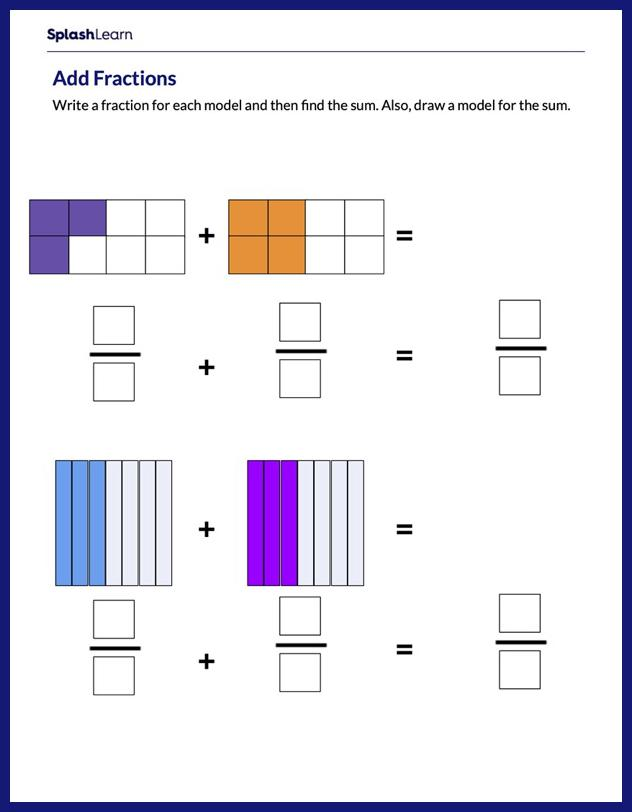## Area Model Division with a Remainder

Sometimes, you’ll want to divide numbers that don’t divide evenly from one another. In these cases, it can be helpful to use an area model.

Let’s look at some division area model examples.

Divide 443 by 4 using area model division.

Step 1: Let’s break the numbers into 400, 40, and 3.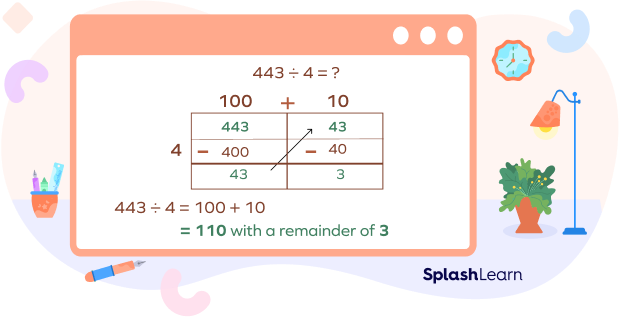Step 2: Dividing 400 by 4, we get 100,

40 divided by 4 gives us 10,

Since 3 cannot be divided by 4, it will be our remainder.

Step 3: Now, we need to add 100 and 10 together to get a quotient, which gives us 110.

So, our final product is quotient $= 110$, remainder $= 3$.

## Area Model for Dividing Decimal Numbers

Dividing decimal numbers can be challenging, but it doesn’t have to be. When you know how to use a few tricks like reducing decimals or using an area model, dividing decimals becomes much easier. Let’s learn the steps through area model division problems.

Divide 225.5 by 5 using area model

Step 1: We can divide the decimal numbers exactly like the regular ones by removing the decimal and adding it in the final step.

So instead of dividing 225.5 by 5, we will divide 2255 by 5.

Step 2: Now, let’s break them into easily divisible numbers, which are: 2000, 200, 50, and 5.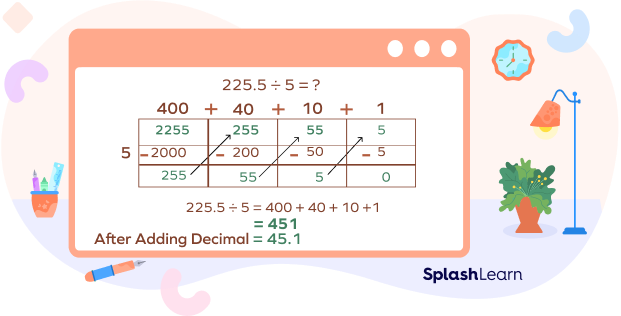Step 3: Now, let’s divide each by 5:

2000 divided by 5 is 400

200 divided by 5 is 40

50 divided by 5 is 10

5 divided by 5 is 1

Step 4: Now, let’s add them together: $400 + 40 + 10 + 1$, giving us 451.

Step 5: Finally, let’s add the decimal we removed earlier. So, 451 will become 45.1, which is our final quotient.

1. It makes it easier to divide fractions or decimal numbers by multiplying them instead of trying to use long division with both parts.

2. It is a good way to help students remember how subtraction or “take away” works.

3. It helps students solve long divisions easily and also helps them understand the process of dividing numbers.

1. Divide 728 by 14 using the area model.

Solution : Let’s start by breaking down 728 into 700 and 28.

Dividing 700 by 14 gives us 50, and 28 divided by 14 is 2.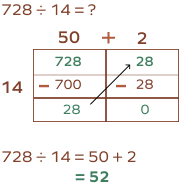Now, let’s add these two to get the quotient $(50 + 2)$, which gives us 52.

2. Divide 624 by 3 using the area model of division.

Solution : Let’s start by breaking down 624, which gives us 600 and 24.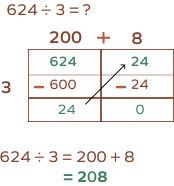600 divided by 3 gives us 200, and 24 divided by 3 gives us 8.

On adding the two, we get 208.

3. Divide 3213 by 4 using the area model of division.

Solution : Let’s start by breaking down 3214, which gives us 3200 and 14.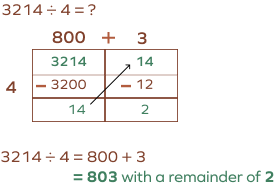3200 divided by 4 gives us 800,

14 divided by 4 gives us 3, with a remainder of 2.

So, the final answer is 803, with a remainder of 2.

4. What is the quotient when you divide 4977 by 7 using area model?

Solution : First, let’s break down 4977 into 4900 and 77.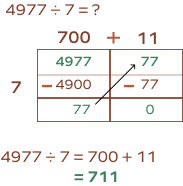Dividing 4900 by 7, we get 700,

and 77 divided by 7 gives us 11

Adding these numbers $(700 + 11)$ gives us 711

So, the final answer is 711.

Attend this quiz & Test your knowledge.

## What is the quotient when you divide 345 by 5 using the area model?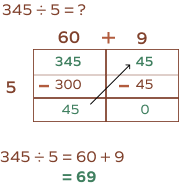## What is the answer when you divide 246 by 4 using the area model?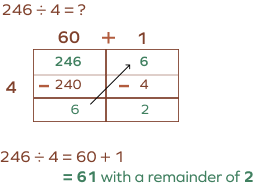## What is the quotient when you divide 29.7 by 3 using the area model?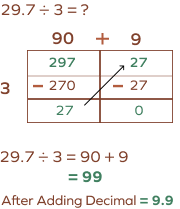## What is the quotient when you divide 69.20 by 4 using the area model?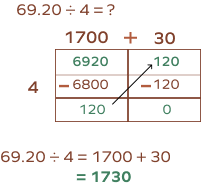Can area model division be used for fractions?

Yes, you can efficiently use area model division to divide fractions.

Can the area model be used for multiplication?

Yes, you can use area models to solve a multiplication problem with large numbers. The multiplicands will be the length and breadth of a rectangle, and their product will be the area of the rectangle.

To find the area, you divide the rectangle into smaller segments. The sum of the areas of the smaller rectangles gives you the total area, which will be the product. This method is also called box multiplication.

What is the purpose of the area model division?

Area model division helps students visualize and make longer division problems easier to solve.

Why is the area model for division important?

Division using area models gives students another perspective on math equations, which is extremely valuable since not all kids (or adults) think in the same way.

## RELATED POSTS

• Area in Math – Definition, Composite Figures, FAQs, Examples
• Seconds to Minutes Conversion
• Bar Graph – Definition, Types, Examples, Practice Problems, Facts
• Decimal Point – Definition, Place Value Chart, Examples, Facts
• Divisor – Definition, Examples, Practice Problems, FAQs
• Kindergarten
• Arts & Music
• English Language Arts
• World Language
• Social Studies - History
• Special Education
• Holidays / Seasonal
• Independent Work Packet
• Easel by TPT## Easel Activities## Easel Assessments

division area model worksheets

## All Formats

Resource types, all resource types, results for division area model worksheets.

• Price (Ascending)
• Price (Descending)
• Most Recent## Division Worksheets using Area Model## Area Model Division Worksheets• Easel Activity## Partial Quotients and Area Model Division Worksheets (1-Digit Divisors)## Area Model Division Practice Worksheets## Division Drawings Rectangular Area Model for Long Division ( Worksheets )(Games)## 3 Digit by 1 Digit Area Model Division With and Without Remainders Worksheets## 4.NBT.6: 2 x 1 Digit Division Using the Area Model Math Video and Worksheet## Division Area Model Worksheet## 4.NBT.6: 3 x 1 Digit Area Model Division Math Video and Worksheet## Division Area Model Worksheets## Free Area Model / Box Method Division Worksheets | 2 Digit & 3 Digit by 1 Digit## Area Model , Partial Quotients & Long Division Worksheets | 1 & 2-Digit Divisors## 5.NBT.6: 3 x 2 Digit Division Using Area Model Math Video and Worksheet## 2 Digit by 1 Digit Area Model Division Worksheets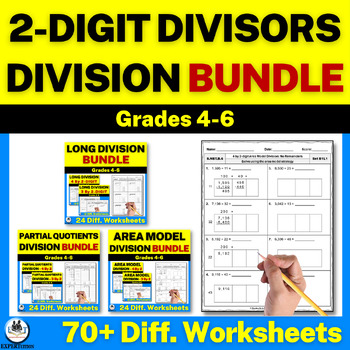## 2-Digit Divisors Area Model , Partial Quotients & Long Division Worksheets Bundle## 4 Digit by 1 Digit Area Model Division With and Without Remainders Worksheets## 5.NBT.6: 4 x 2 Digit Division Using Area Model Math Video and Worksheet## 4.NBT.6: 4 x 1 Digit Area Model Division Math Video and Worksheet## Area Model Division With Remainders and No Remainders Worksheets Big Bundle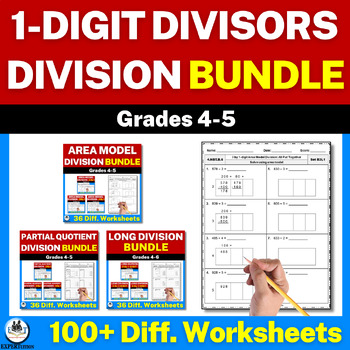## 1-Digit Divisors Area Model , Partial Quotients & Long Division Worksheets Bundle## 2-Digit Divisors Area Model Division With & Without Remainders Worksheets Bundle## 4 Digit by 2 Digit Area Model Division With and Without Remainders Worksheets## 3 Digit by 2 Digit Area Model Division With and Without Remainders Worksheets## NUMBER NOTES Array & Area Model Division Activity ★ 4th Grade Worksheets• We're Hiring
• Help & FAQ
• Student Privacy#### IMAGES

1. MATH G4: Solve Division Problems With Remainders Using Arrays And Area Models2. Solving Division Using Area Model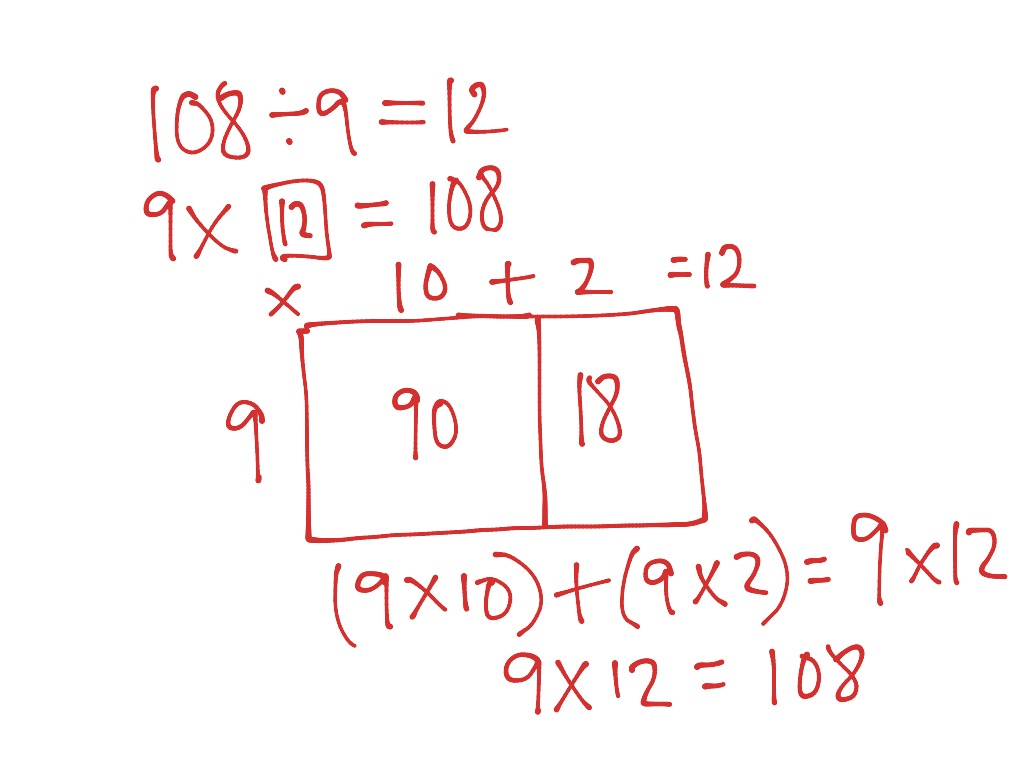3. Division With Remainders Worksheet4. Division5. Math Worksheets 3rd-4th Grade. Solve division word problems with remainders. Learn to interpret6. Can We Solve Remainder Problems Without Long Division?#### VIDEO

1. Division Remainder

2. Algebra Long Division an Explanation

3. Long Division with no Remainders

4. long division problem

5. math primary 5 using area model to divide

6. Demystifying Division: Unveiling the Strategy of Partial Quotients

1. What Is an Area Model?

An area model is a graphical representation of a multiplication or division problem. Area models are used in math to help students better visualize what is happening in a problem, creating a conceptual understanding of often abstract proble...

2. What Are the Six Steps of Problem Solving?

The six steps of problem solving involve problem definition, problem analysis, developing possible solutions, selecting a solution, implementing the solution and evaluating the outcome. Problem solving models are used to address issues that...

3. What Are the Factors of 48?

The factors of 48 include 1, 2, 3, 4, 6, 8, 12, 16, 24 and 48. The number 48 is divisible by each of the factors without any remainder being left over. Finding the factors of a whole number requires some trial and error in the form of a ser...

4. Solve division problems without remainders using the area model

divide? Lesson 20. NYS. COMMON CORE MATHEMATICS CURRICULUM. 4. •. 3. Lesson 20. : Solve division problems without remainders using the area model.

5. Division using the Area Model

How to solve division problems without remainders using the area model, examples and step by step solutions, Draw a number bond and use the distributive

6. Solve division problems without remainders: using multiplication rules

Solve division problems without remainders: using multiplication rules In ... whole number using arrays, area models, or equations; TEKS.3.4.

7. Division Using Area Models

In this video I explain the Area Model method of division. As high school math is my specialty, I should say I "attempt" to explain this

8. Lesson 21 Homework 4•3

Solve division problems with remainders using the area model. Date: 8/28/13. 3.E.97. © 2013 Common Core, Inc. Some rights reserved. commoncore.org.

9. Division with area models (video)

Use your math skills while using the other things you,ve got. 3 comments

10. 4.3.E.20

Tony drew the following area model to find an unknown length. What division equation did he model? visibilityView Drawing. 4.NBT.6. 10. 2. Solve 42 ÷ 3

11. Solve 87 ÷ 5 by using an area model. Use long division and the

Students develop an understanding of remainders. They use different methods to solve division problems. You can expect to see homework that asks your child to

12. What Is Area Model Division? Definition, Examples, Facts

Understand the meaning of area model division and learn how to apply the area model for division of numbers with and without remainder.

13. Results for division area model worksheets

Browse division area model worksheets resources on Teachers Pay ... Digit Area Model Division With and Without Remainders Worksheets.

14. Lesson 19 Homework 4.3